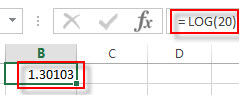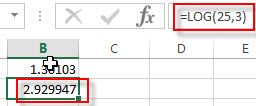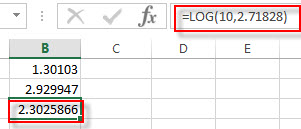# Excel LOG Function

This post will guide you how to use Excel LOG function with syntax and examples in Microsoft excel.

### Description

The Excel LOG function returns the logarithm of a given number based on a supplied base. And the default base argument is 10.

The LOG function is a build-in function in Microsoft Excel and it is categorized as a Math and Trigonometry Function.

The LOG function is available in Excel 2016, Excel 2013, Excel 2010, Excel 2007, Excel 2003, Excel XP, Excel 2000, Excel 2011 for Mac.

### Syntax

The syntax of the LOG function is as below:

`= LOG (number,[base])`

Where the LOG function arguments are:

• number – This is a required argument. The number that you want the logarithm. And the number must be a positive real number.
• Base – This is an optional argument. The base of the logarithm. If the base is omitted, the base argument will be set to 10.

Note:

• If either the supplied number argument or base argument is negative or zero, the LOG function will return #NUM! Error.
• If the base argument is equal to 1, the LOG function will return #DIV/0! Error.
• If the number argument or the base argument is non-numeric, the LOG function will return #VALUE! Error.

### Excel LOG Function Examples

The below examples will show you how to use Excel LOG Function to calculate the logarithm of a given number, to a supplied base.

1# to get the logarithm of 20, and using the default base argument, enter the following formula in Cell B1.

`= LOG(20)`2# to get the logarithm of 25 with base 3, enter the following formula in Cell B2.

`=LOG(25,3)`3# to get the logarithm of 10 with base e (2.71828), enter the following formula in Cell B2.

`=LOG(10,2.71828)`### Related Functions

• Excel LOG10 function
The Excel LOG10 function returns the base-10 logarithm of a given number.The syntax of the LOG10 function is as below:= LOG10 (number)…
• Excel LN function
The Excel LN function returns the natural logarithm of a given number.  And the natural logarithms are based on the constant e (the value is equal to 2.718281828…). The syntax of the LN function is as below:= LN (number)…